### MODEL Statement

MODEL dependents = regressors </ options> ;

The MODEL statement names the dependent variables and the independent variables. Variables specified in the MODEL statement must be numeric variables in the data set being analyzed.

Table 53.2 summarizes the options available in the MODEL statement.

Table 53.2: Summary of MODEL Statement Options

Option

Description

Fit Options

specifies the number of points in k-d tree buckets

specifies the degree of local polynomials (1 or 2)

specifies the method of computing lookup degrees of freedom

specifies direct fitting at every data point

specifies the variables whose squares are to be dropped from local quadratic polynomials

specifies the interpolating polynomials (linear or cubic)

specifies the number of reweighting iterations

specifies the method used to scale the regressor variables

specifies that automatic smoothing parameter selection be done

specifies the list of smoothing values

Output Statistics Table Options

requests CLM, RESIDUAL, SCALEDINDEP, STD, and T options

displays confidence limits for mean predictions

displays residuals

displays scaled independent variable coordinates

displays standard errors of the mean predicted values

displays t statistics

Other options

sets significance level for confidence intervals

specifies which tables are to be displayed

displays the trace of the smoothing matrix

The following options are available in the MODEL statement after a slash (/).

ALL

requests all these options: CLM, RESIDUAL, SCALEDINDEP, STD, and T.

ALPHA=number

sets the significance level used for the construction of confidence intervals for the current MODEL statement. The value must be between 0 and 1; the default value of 0.05 results in 95% intervals.

BUCKET=number

specifies the maximum number of points in the leaf nodes of the k-d tree. The default value used is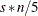, where s is a smoothing parameter value specified using the SMOOTH= option and n is the number of observations being used in the current BY group. The BUCKET= option is ignored if the DIRECT option is specified.

CLM

requests that% confidence limits on the mean predicted value be added to the Output Statistics table. By default, 95% limits are computed; the ALPHA= option in the MODEL statement can be used to change the significance level. The use of this option implicitly selects the model option DFMETHOD=EXACT if the DFMETHOD= option has not been explicitly used.

DEGREE=1 | 2

sets the degree of the local polynomials to use for each local regression. The valid values are 1 for local linear fitting and 2 for local quadratic fitting, with 1 being the default.

DETAILS <( tables )>

selects which tables to display, where tables is one or more of the specifications KDTREE, MODELSUMMARY, OUTPUTSTATISTICS, and PREDATVERTICES:

• KDTREE displays the k-d tree structure.

• MODELSUMMARY displays the fit criteria for all smoothing parameter values that are specified in the SMOOTH= option in the MODEL statement, or that are fit with automatic smoothing parameter selection.

• OUTPUTSTATISTICS displays the predicted values and other requested statistics at the points in the input data set.

• PREDATVERTICES displays fitted values and coordinates of the k-d tree vertices where the local least squares fitting is done.

The KDTREE and PREDATVERTICES specifications are ignored if the DIRECT option is specified in the MODEL statement. Specifying the option DETAILS with no qualifying list outputs all tables.

DFMETHOD=NONE | EXACT | APPROX <(approx-options)>

specifies the method used to calculate the lookup degrees of freedom used in performing statistical inference. The default is DFMETHOD=NONE, unless you specify any of the MODEL statement options ALL, CLM, STD, and T, or any SCORE statement CLM option, in which case the default is DFMETHOD=EXACT.

You can specify the following approx-options in parentheses after the DFMETHOD=APPROX option:

QUANTILE=number

specifies that the smallest 100(number)% of the nonzero coefficients in the smoothing matrix be set to zero in computing the approximate lookup degrees of freedom. The default value is QUANTILE=0.9.

CUTOFF=number

specifies that coefficients in the smoothing matrix whose magnitude is less than the specified value be set to zero in computing the approximate lookup degrees of freedom. Using the CUTOFF= option overrides the QUANTILE= option.

See the section Sparse and Approximate Degrees of Freedom Computation for a description of the method used when the DFMETHOD=APPROX option is specified.

DIRECT

specifies that local least squares fits are to be done at every point in the input data set. When the direct option is not specified, a computationally faster method is used. This faster method performs local fitting at vertices of a k-d tree decomposition of the predictor space followed by blending of the local polynomials to obtain a regression surface.

DROPSQUARE=(variables)

specifies the quadratic monomials to exclude from the local quadratic fits. This option is ignored unless the DEGREE=2 option has been specified.

For example,

model z=x y / degree=2 dropsquare=(y)


uses the monomials 1, x, y,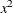, and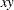in performing the local fitting.

INTERP=LINEAR | CUBIC

specifies the degree of the interpolating polynomials used for blending local polynomial fits at the k-d tree vertices. This option is ignored if the DIRECT option is specified in the model statement. INTERP=CUBIC is not supported for models with more than two regressors. The default is INTERP=LINEAR.

ITERATIONS=number

specifies the total number of iterations to be done. The first iteration performs an initial LOESS fit. Subsequent iterations perform iterative reweighting. Such iterations are appropriate when there are outliers in the data or when the error distribution is a symmetric long-tailed distribution. The default number of iterations is 1.

RESIDUAL | R

specifies that residuals be included in the Output Statistics table.

SCALE=NONE | SD < (number) >

specifies the scaling method to be applied to scale the regressors. The default is NONE, in which case no scaling is applied. A specification of SD(number) indicates that a trimmed standard deviation is to be used as a measure of scale, where number is the trimming fraction. A specification of SD with no qualification defaults to 10% trimmed standard deviation.

SCALEDINDEP

specifies that scaled regressor coordinates be included in the output tables. This option is ignored if the SCALE= model option is not used or if SCALE=NONE is specified.

SELECT=criterion <( <GLOBAL> <PRESEARCH> <STEPS> <RANGE(lower,upper)> )>
SELECT=DFCriterion <( target <GLOBAL> <PRESEARCH> <STEPS> <RANGE(lower,upper)> )>

specifies that automatic smoothing parameter selection be done using the named criterion or DFCriterion. Valid values for the criterion are as follows:

AICC

specifies the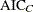criterion (Hurvich, Simonoff, and Tsai, 1998).

AICC1

specifies the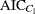criterion (Hurvich, Simonoff, and Tsai, 1998).

GCV

specifies the generalized cross validation criterion (Craven and Wahba, 1979).

The DFCriterion specifies the measure used to estimate the model degrees of freedom. The measures implemented in PROC LOESS all depend on prediction matrixrelating the observed and predicted values of the dependent variable. Valid values for the DFCriterion are as follows:

DF1

specifies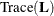.

DF2

specifies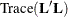.

DF3

specifies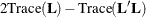.

For both types of selection, the smoothing parameter value is selected to yield a minimum of an optimization criterion. If you specify criterion as one of AICC, AICC1, or GCV, the optimization criterion is the specified criterion. If you specify DFCriterion as one of DF1, DF2, or DF3, the optimization criterion is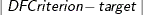, where target is a specified target degree of freedom value. Note that if you specify a DFCriterion, then you must also specify a target value. See the section Automatic Smoothing Parameter Selection for definitions and properties of the selection criteria.

The selection is done as follows:

• If you specify the SMOOTH=value-list option, then PROC LOESS selects the largest value in this list that yields the global minimum of the specified optimization criterion.

• If you do not specify the SMOOTH= option, then PROC LOESS finds a local minimum of the specified optimization criterion by using a golden section search of values less than or equal to one.

You can specify the following suboptions in parentheses after the specified criterion to alter the behavior of the SELECT= option:

GLOBAL

specifies that a global minimum be found within the range of smoothing parameter values examined. This suboption has no effect if you also specify the SMOOTH= option in the MODEL statement.

PRESEARCH

requests an initial grid search to find a smoothing parameter range within which the subsequent golden section search is done. The initial point in this grid is the smoothing parameter value corresponding to the smallest number of points, n, in the local neighborhoods that yields a fit that does not interpolate all the data points. Subsequent fits with number of local points n + 1, n + 2, n + 4, n + 8, ... are evaluated until either the number of local points exceeds the number of fitting points or the SELECT=criterion starts increasing. This suboption is ignored if you additionally specify the GLOBAL suboption of the SELECT= option or if you specify the SMOOTH= option in the MODEL statement. If you additionally specify the RANGE= suboption, then the golden section search is done on the intersection of the range found by this grid search and the range that you specify in the RANGE= suboption. This option is useful for data exhibiting features at multiple scales, because in such cases the SELECT= criterion often has multiple local minima. Using the PRESEARCH option increases the likelihood that the golden section search will find the global minimum of the SELECT= criterion. See Example 53.4 for such an example.

RANGE(lower,upper)

specifies that only smoothing parameter values greater than or equal to lower and less than or equal to upper be examined.

STEPS

specifies that all models evaluated in the selection process be displayed.

For models with one dependent variable, if you specify neither the SELECT= nor the SMOOTH= options in the MODEL statement, then PROC LOESS uses SELECT=AICC.

The following table summarizes how the smoothing parameter values are chosen for various combinations of the SMOOTH= option, the SELECT= option, and the SELECT= option modifiers.

Table 53.3: Smoothing Parameter Value(s) Used for Combinations of SMOOTH= and SELECT= OPTIONS for Models with One Dependent Variable

Syntax

Search Method

Search Domain

default

golden section using AICC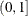SMOOTH=list

no selection

values in list

SMOOTH=list SELECT=criterion

global

values in list

SMOOTH=list SELECT=criterion ( RANGE(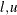) )

global

values in list within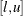SELECT=criterion

golden sectionSELECT=criterion ( RANGE(l,u) )

golden sectionSELECT=criterion ( GLOBAL )

globalSELECT=criterion ( GLOBAL RANGE() )

globalSome examples of using the SELECT= option follow:

SELECT=GCV

specifies selection that uses the GCV criterion.

SELECT=DF1(6.3)

specifies selection that uses the DF1 DFCriterion with target value 6.3.

SELECT=AICC(STEPS)

specifies selection that uses the AICC criterion, showing all step details.

SELECT=DF2(7 GLOBAL)

specifies selection that uses a global search algorithm to find the smoothing parameter that yields the DF2 DFCriterion closest to the target value 7.

Note: The SELECT= option cannot be used for models with more than one dependent variable.

SMOOTH=value-list

specifies a list of positive smoothing parameter values. If you do not specify the SELECT= option in the MODEL statement, then a separate fit is obtained for each SMOOTH= value specified. If you do specify the SELECT= option, then models with all values specified in the SMOOTH= list are examined, and PROC LOESS selects the value that minimizes the criterion specified in the SELECT= option.

For models with two or more dependent variables, if the SMOOTH= option is not specified in the MODEL statement, then SMOOTH=0.5 is used as a default.

STD

specifies that standard errors of the mean predicted values be included in the Output Statistics table. The use of this option implicitly selects the model option DFMETHOD=EXACT if the DFMETHOD= option has not been explicitly used.

T

specifies that t statistics are to be included in the Output Statistics table. The use of this option implicitly selects the model option DFMETHOD=EXACT if the DFMETHOD= option has not been explicitly used.

TRACEL

specifies that the trace of the prediction matrix as well as the GCV and AICC statistics be included in the Fit Summary table. The use of any of the MODEL statement options ALL, CLM, DFMETHOD=EXACT, DIRECT, SELECT=, STD, and T implicitly selects the TRACEL option.